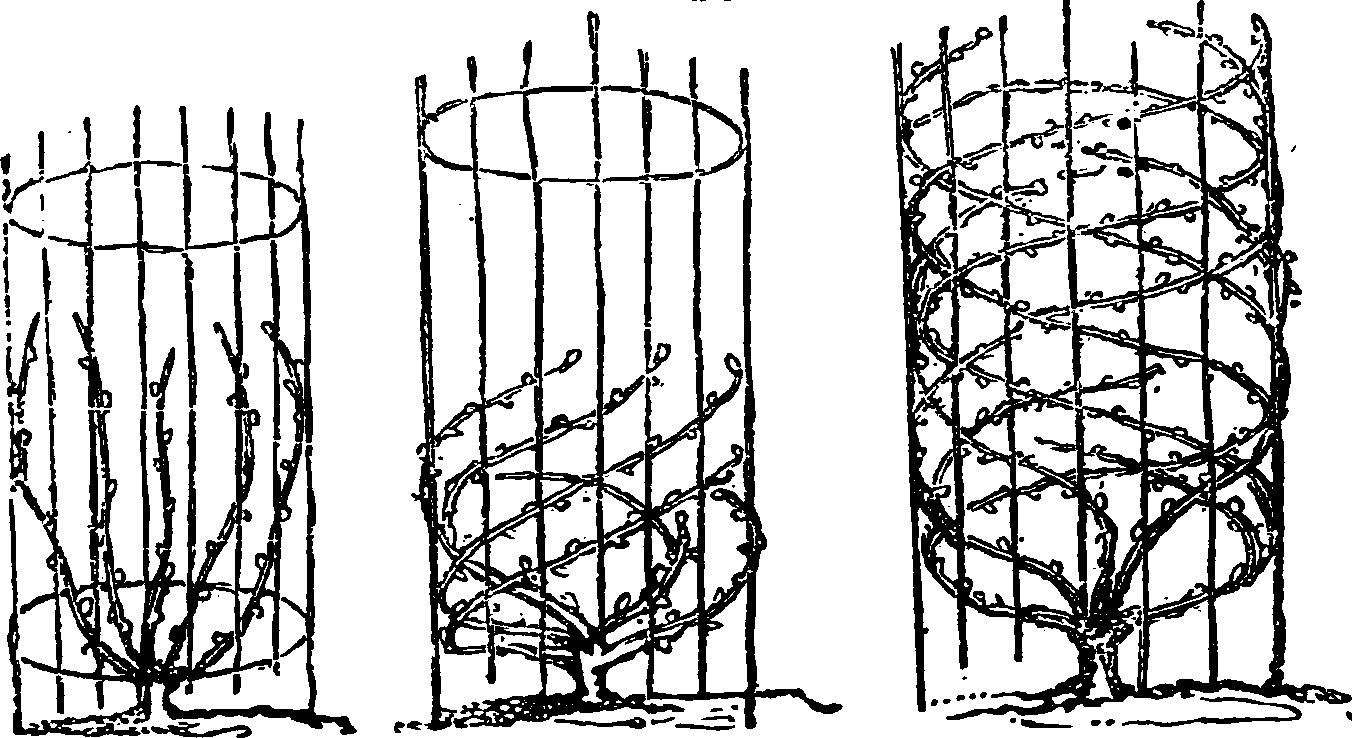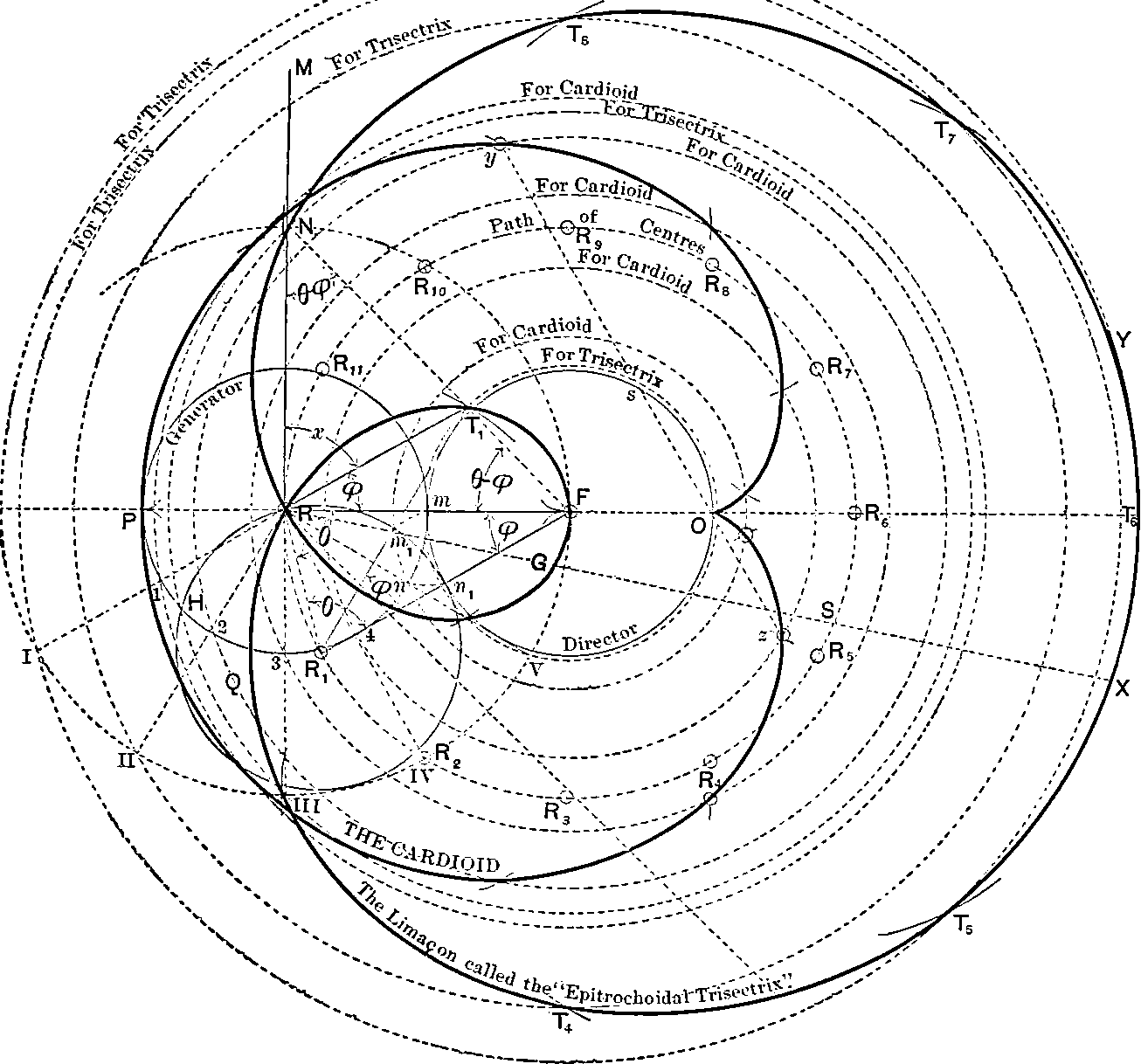# Algebra I would like to learn## Stringology

Long story. Group theory for languages and automata.

• Properties of the free group. (because of the stringology thing)
• Cayley Graphs. (because of the stringology thing)

## Probabilistic

Probabilistic methods in algebra (as opposed to algebraic methods in probability).

### Cycles in a random permuation

The number of cycles in a random permutation

Let $$n$$ be a natural number, and let $$\sigma: \{1,\ldots,n\} \rightarrow \{1,\ldots,n\}$$ be a permutation of $$\{1,\ldots,n\}$$, drawn uniformly at random. Using the cycle decomposition, one can view $$\sigma$$ as the disjoint union of cycles of varying lengths (from $$1$$ to $$n$$). For each $$1 \leq k \leq n$$, let $$C_k$$ denote the number of cycles of $$\sigma$$ of length $$k$$; thus the $$C_k$$ are natural number-valued random variables with the constraint $\sum_{k=1}^n k C_k = n.$ We let $$C := \sum_{k=1}^n C_k$$ be the number of cycles (of arbitrary length); this is another natural number-valued random variable, of size at most $$n$$… for fixed $$1 \leq k_1 < \ldots < k_r,\, C_{k_1},\ldots,C_{k_r}$$ converge in joint distribution to $$r$$ independent Poisson distributions of intensity $$\frac{1}{k_1},\ldots,\frac{1}{k_r}$$ respectively…

### Large prime factors of a random number

Terry Tao uses Poisson-Dirichlet process in a fun way

Define a partition of $$1$$ to be a finite or infinite multiset $$\Sigma$$ of real numbers in the interval $$I \in (0,1]$$ (that is, an unordered set of real numbers in $$I$$, possibly with multiplicity) whose total sum is $$1: \sum_{t \in \Sigma}t = 1$$. For instance, $$\{1/2,1/4,1/8,1/16,\ldots\}$$ is a partition of $$1$$… In the spirit of the universality phenomenon, one can ask what is the natural distribution for what a “typical” partition should look like; thus one seeks a natural probability distribution on the space of all partitions, analogous to (say) the Gaussian distributions on the real line, or GUE distributions on point processes on the line, and so forth. It turns out that there is one natural such distribution which is related to all three examples above, known as the Poisson-Dirichlet distribution.

## Connection to symmetries